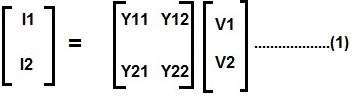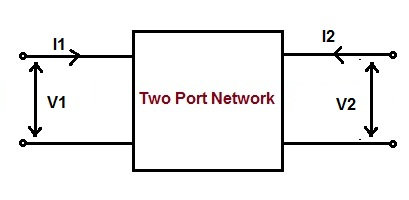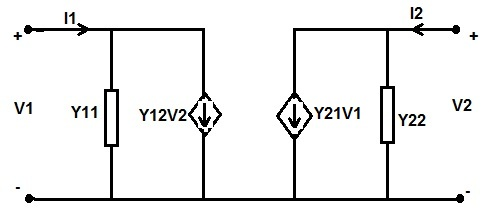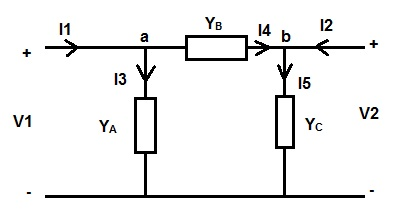# Y Parameter of Two Port Network

Y parameter of two port network is a 2×2 admittance matrix. Since admittance is the ratio of circuit current and voltage, therefore this admittance matrix gives the relationship between the input and output current and voltage of the network. It is also known as short circuit admittance parameter.

The Y parameter for a two port network is defined as

[ I ] = [ Y ] [ V ]

where [ Y ] is the admittance matrix, [ I ] and [ V ] are the current and voltage matrix.From the above matrix form representation of two port network, it is clear that there are four admittance parameters i.e. Y11, Y12, Y21 and Y22. Each of them has a special meaning and significance which will be discussed latter in this post.

### Calculation of Y Parameter:

Let us consider a two port network. Let V1, I1, V2 and I2 are the input voltage, input current, output voltage and output current respectively.The relationship between the input and output quantities for the above network is obtained using (1) as below.

I1 = Y11V1 + Y12V2 ……(2)

I2 = Y21V1 + Y22V2 …….(3)

Assuming the output of the two port network to be short circuited, therefore

V2 = 0

Now putting V2 = 0 in (2), we get

I1 = Y11V1

Y11 = (I1 / V1)

Similarly putting V2 = 0 in (3), we get

I2 = Y21V1

Y21 = (I2 / V1)

Again assuming input port of the two port network to be short circuited, the input voltage will be zero.

V1 = 0

Now putting V1 = 0 in (2), we get

I1 = Y12V2

Y12 = (I1 / V2)

Similarly putting V1 = 0 in (3), we get

I2 = Y22V2

Y22 = (I2 / V2)

Thus there are four Y parameter for a two port or four terminal network. Their values are tabulated below.

 Y11 (I1 / V1) Condition: Output port of the two port network is short circuited i.e. V2 = 0 Y21 (I2 / V1) Y12 (I1 / V2) Condition: Input port of the two port network is short circuited i.e. V1 = 0 Y22 (I2 / V2)

### Significance of Different Y Parameter:

• Since Y11 is the ratio of input current and voltage when the output port is short circuited, therefore it is known as input driving point admittance.
• Y22 is the ratio of output current and voltage when input port is short circuited, therefore it is called output driving point admittance of the network.
• Y12 is the ratio of input current and output voltage when input port is short circuited, therefore it is called reverse transfer admittance.
• Y21 is the ratio of output current and input voltage when output port is short circuited, therefore it is called forward transfer admittance.

### Equivalent Circuit Representation of Y Parameter:

The equivalent circuit of Y parameter for two port network can be represented using (2) and (3) as shown below.In the above circuit, the current sources Y12V2 and Y21V1 are called Voltage Controlled Current Source.

Y Parameter Solved Problems:

To better understand the discussed concept of Y parameter, we will consider one example.

Example:

Find the Y parameter for the π circuit given below.Solution:

Applying Kirchoff’s Current Law at node ‘a’,

I1 = I3 + I4

= V1YA + (V1 – V2)YB

= (YA + YB)V1 + (-YB)V2

Thus, I1 = (YA + YB)V1 + (-YB)V2

But from (2),

I1 = Y11V1 + Y12V2

Comparing the above two expressions, we get

Y11 = (YA + YB)

Y12 = -YB

Again, applying Kirchoff’s Current Law at node ‘b’

I2 = I5 – I4

= YCV2 – (V1 – V2)YB

= (-YB)V1 + (YB + YC)V2

Thus,

I2 = (-YB)V1 + (YB + YC)V2

But from (3),

I2 = Y21V1 + Y22V2

Comparing the above two expressions, we get

Y22 = (YA + YB)

Y21 = -YB

Hence, the four Y parameters are

Y11 = (YA + YB), Y12 = -YB, Y22 = (YA + YB), Y21 = -YB  (Answer)

### One thought on “Y Parameter of Two Port Network”

1.Yogesh Sambare says: